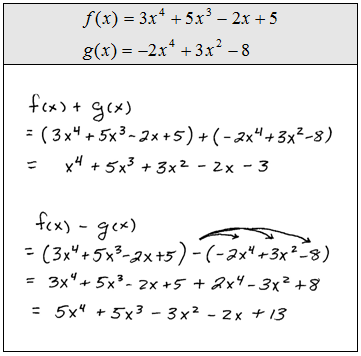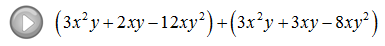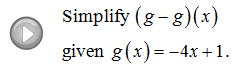## Algebra

Showing posts with label polynomials. Show all posts
Showing posts with label polynomials. Show all posts

Adding polynomials requires us to first identify then combine like terms.

Remember that like terms are terms with the exact same variable part.When subtracting polynomials you distribute the negative one first and then identify and combine like terms.

Subtract the polynomials.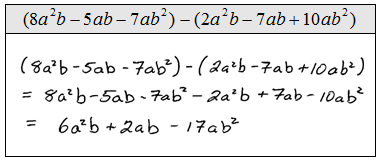We may encounter function notation,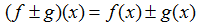In this case, just add or subtract the given polynomials as we did in the above examples.

For the given functions find (f + g)(x) and (f - g)(x).